Loss of economic efficiency when the optimal outcome is not achieved

Deadweight loss refers to the loss of economic efficiency when the equilibrium outcome is not achievable or not achieved. In other words, it is the cost born by society due to market inefficiency.

### Video Explanation of Deadweight Loss

Below is a short video tutorial that describes what deadweight loss is, provides the causes of deadweight loss, and gives an example calculation.

• Price floors: The government sets a limit on how low a price can be charged for a good or service. An example of a price floor would be minimum wage.
• Price ceilings: The government sets a limit on how high a price can be charged for a good or service. An example of a price ceiling would be rent control – setting a maximum amount of money that a landlord can collect for rent.
• Taxation: The government charging above the selling price for a good or service. An example of taxation would be a cigarette tax.

### Imperfect Competition and Deadweight Loss

Deadweight loss also arises from imperfect competition such as oligopolies and monopolies. In imperfect markets, companies restrict supply to increase prices above their average total cost. Higher prices restrict consumers from enjoying the goods and, therefore, create a deadweight loss.

Imagine that you want to go on a trip to Vancouver. A bus ticket to Vancouver costs \$20, and you value the trip at \$35. In this situation, the value of the trip (\$35) exceeds the cost (\$20) and you would, therefore, take this trip. The net value that you get from this trip is \$35 – \$20 (benefit – cost) = \$15.

Prior to buying a bus ticket to Vancouver, the government suddenly decides to impose a 100% tax on bus tickets. Therefore, this would drive the price of bus tickets from \$20 to \$40. Now, the cost exceeds the benefit; you are paying \$40 for a bus ticket from which you only derive \$35 of value. In this scenario, the trip would not happen and the government would not receive any tax revenue from you. The deadweight loss is the value of the trips to Vancouver that do not happen because of the tax imposed by the government.

Consider the graph below: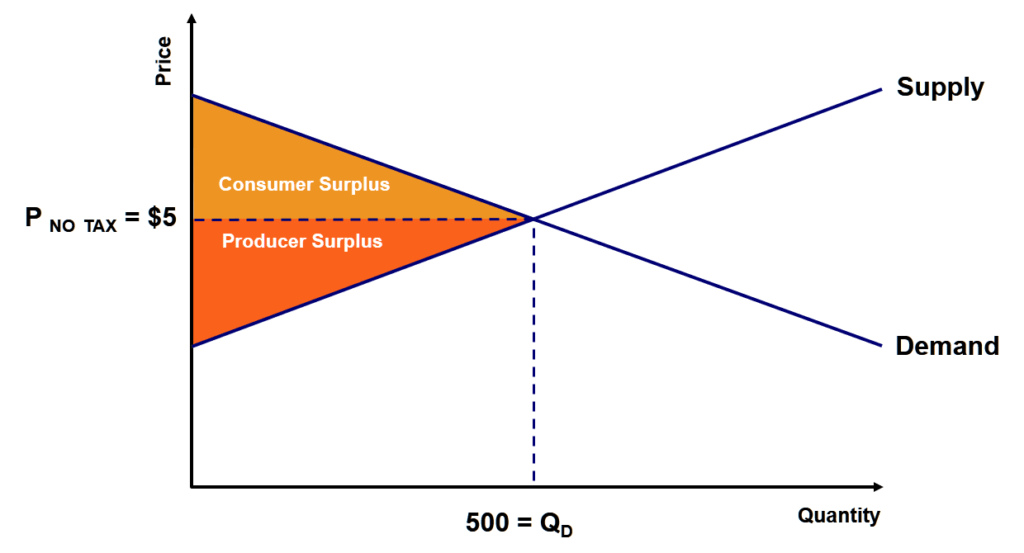At equilibrium, the price would be \$5 with a quantity demand of 500.

• Equilibrium price = \$5
• Equilibrium demand = 500

In addition, regarding consumer and producer surplus:

• Consumer surplus is the consumer’s gain from an exchange. The consumer surplus is the area below the demand curve but above the equilibrium price and up to the quantity demand.
• Producer surplus is the producer’s gain from exchange. The producer surplus is the area above the supply curve but below the equilibrium price and up to the quantity demand.

Let us consider the effect of a new after-tax selling price of \$7.50: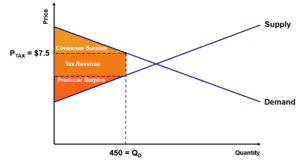The price would be \$7.50 with a quantity demand of 450. Taxes reduce both consumer and producer surplus. However, taxes create a new section called “tax revenue.” This is the revenue collected by governments at the new tax price.

With this new tax price, there would be a deadweight loss: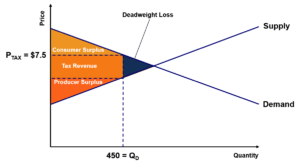As illustrated in the graph, deadweight loss is the value of the trades that are not made due to the tax. The blue area does not occur because of the new tax price. Therefore, no exchanges take place in that region, and deadweight loss is created.

To figure out how to calculate deadweight loss from taxation, refer to the graph shown below: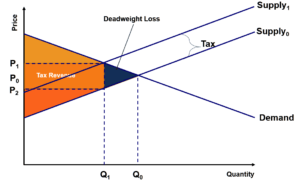#### Notes:

• The equilibrium price and quantity before the imposition of tax is Q0 and P0.
• With the tax, the supply curve shifts by the tax amount from Supply0 to Supply1. Producers would want to supply less due to the imposition of a tax.
• The buyer’s price would increase from P0 to P1 and the seller would receive a lower price for the good from P0 to P2.
• Due to the tax, producers supply less from Q0 to Q1.

The deadweight loss is represented by the blue triangle and can be calculated as follows: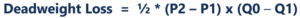### More Resources

CFI is a global provider of the Financial Analyst Certificate in valuation modeling and financial analysis. To learn more, explore these additional CFI resources below:

• Fiscal Policy
• Normative Economics